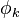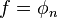Difference between revisions of "User:IssaRice/Computability and logic/Function versus algorithm"

If we want to enumerate all of the computable partial functions (say, in order to do some diagonalization argument) the usual way to do this is to enumerate all of the algorithms first, encode the algorithm as an index, and then use this enumeration as an enumeration of the computable partial functions. What this means is that when we refer to some computable partial function as$\phi_k$, for example, then actually we know more than just what the function is: we also know that$k$ is an integer that encodes the algorithm that computes$\phi_k$. In contrast, if we just had some computable partial function called$f$, then we know that some algorithm computes$f$, but we have no name for it/a canonical choice for it, so we have to pick some number, say,$n$, so that$f = \phi_n$.